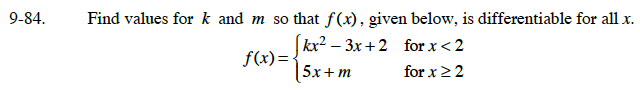### Home > CALC > Chapter 9 > Lesson 9.3.1 > Problem9-84

9-84.

Find values for k and m so that f (x) , given below, is differentiable for all x. Homework Help ✎$kx^2-3x+2=5x+m\text{ when }x=2$

AND